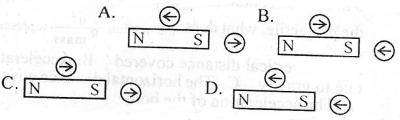### Physics Past Questions

3751

Which of the following statements about magnetic lines of force is not correct? They

• A. pass through even non-magnetic materials
• B. run from the north pole to the south pole
• C. are in a state of tension and tend to be shorted
• D. intersect one another
3752

In which of the following activities is an induction coil not useful?

• A. Investigation of high voltages
• B. Study of electric discharge
• C. Operation of X-ray tubes
• D. A.C. power transmission
3753

In a series R-L-C circuit, R = 10$$\Omega$$, $$X_{c}$$= 4$$\Omega$$ and $$X_{L}$$ = 9$$\Omega$$. The impedance of the circuit is

• A. 4.1$$\Omega$$
• B. 5.0$$\Omega$$
• C. 10.8$$\Omega$$
• D. 11.2$$\Omega$$
3754A student uses two compass needles to investigate the magnetic field around a bar magnet. Which of the following diagrams show the correct directions of the needles?

• A. A
• B. B
• C. C
• D. D
3755

Which of the following is emitted in a process of natural radioactivity?

• A. $$\alpha$$- particles and $$\beta$$- particles
• B. $$\alpha$$- particles and X-rays
• C. $$\beta$$- particles and X-rays
• D. $$\lambda$$- rays and X-rays